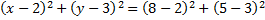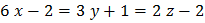# In triangle, equation of sideis. Circumcentre and orthocenter of the triangle are (2, 3) and (5, 8), respectively. Equation of circumcircle of the triangle is a)b)c)d)## Question ID - 55754 :- In triangle, equation of sideis. Circumcentre and orthocenter of the triangle are (2, 3) and (5, 8), respectively. Equation of circumcircle of the triangle is a)b)c)d)3537

(b)Let orthocenter beNow,Also,Hence,andare congruentis image ofin the linewhich isThus, equation of circumcircle isi.e.Next Question :
 The direction cosines of the lineare a)b)c) 1, 2, 3 d) None of these